# Two friends

Two friends met as a good man perish together for a beer. After recovery the most important topics (politics, women, football ...), one asks:
- And how many do you have children?
- I have 3 children.
- And how many years have?
Friend already not want to answer a direct question directly, and then he says:
- The product of their years equals the number 36 and the sum of the years the number of beers we drank here today.
Second to think and says:
- That is not enough!
- You're right, the oldest have bigger ears!

Correct result:

a =  2
b =  3
c =  6

#### Solution:

$b=3$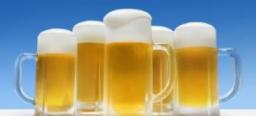We would be pleased if you find an error in the word problem, spelling mistakes, or inaccuracies and send it to us. Thank you!Tips to related online calculators
Do you solve Diofant problems and looking for a calculator of Diofant integer equations?
Do you want to convert time units like minutes to seconds?

## Next similar math problems:

• Write decimalsWrite in the decimal system the short and advanced form of these numbers: a) four thousand seventy-nine b) five hundred and one thousand six hundred and ten c) nine million twenty-six
• Eggs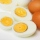One egg boiled in 10 minutes, how long will it take to boil ten eggs at a time?
• Digit sumDetermine for how many integers greater than 900 and less than 1,001 has digit sum digit of the digit sum number 1.
• Prime factorsWrite 98 as product of prime factors
• Divisibility 2How many divisors has integer number 13?
• SickSick Marcel already taken six tablets, which was a quarter of the total number of pills in the pack. How many pills were in the pack?
• Prime factorsFactor the number 6600 into the product of prime numbers.
• Two-digit numberI am a two-digit number less than 20. When I divided by three, then you get the rest 1 and when you divide me by four you get also rest 1. What number am I?
• Bikes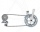Pepa asked Honza how many bikes he had. He replied that he had three-quarters of their total number and another three-quarter of one bike. So how many bikes does Honza have?
• The florist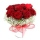The florist had 200 roses in the morning. During the day, more than half sold it. From the remaining roses, it will tie the bouquet. If a bouquet of 3, 4, 5 or 6 roses are bound, one always remains. How many roses from the morning shipment sold?
• Math testIn mathematics, there were 25 problems of three kinds: light 2 points, medium 3 points, heavy 5 points, the best score is 84 points. How many points did Jane have when she solved all the easy examples, half medium and one-third difficult?
• NumberWhat number should be placed instead of the asterisk in number 702*8 to get a number divisible by 6?
• Street numbersLada came to aunt. On the way he noticed that the houses on the left side of the street have odd numbers on the right side and even numbers. The street where he lives aunt, there are 5 houses with an even number, which contains at least one digit number 6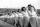Practitioners lined up in rectangle with row with four, five or six exercisers, one always missing to full rectangle. How many exercisers were on the field, if they have estimated not been more than 100?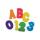Replace the letters A and B by digits so that the resulting number x is divisible by twelve /find all options/. x = 2A3B How many are the overall solutions?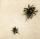In the first basement is more flies than the spiders, the second vice versa. Each basement had spiders and flies together 100 feet. Determine how many could be flies and spiders in the first and second basement. PS. We only need, when you write how many s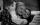The product of the digits of Andrew age as 6 years ago and not equal to 0. Andrew age is also the smallest possible age with this two conditions. After how many years will be the product of the digits of Andrew age again the same as today?